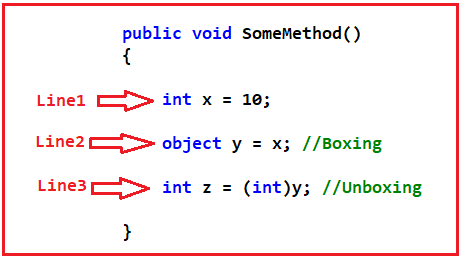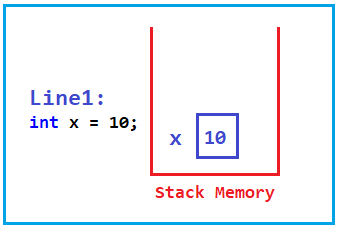# Boxing and Unboxing in C#

## Boxing and Unboxing in C# with Examples

In this article, I am going to discuss Boxing and Unboxing in C# with Examples. Please read our previous article where we discussed Stack, Heap, Value Type, and Reference Type in C# with Examples. The concept of Boxing and Unboxing falls in line with Value Type and Reference Type. So, what we will do in this article is, first we will try to understand Boxing and Unboxing with examples and then we will see how the IL code looks like, and finally we will see the performance implication because of boxing and unboxing in C# application.

##### Boxing and Unboxing in C#:

Let us understand Boxing and Unboxing in C# with an example. Please have a look at the following code.The above method contains three lines of code. Now, let us understand what happens when we execute each line of code.

##### Line1: int x = 10;

When this statement is executed, an integer variable x will be created in the Stack memory with a value of 10. For a better understanding, please have a look at the following diagram.##### Line2: object y = x;

When this statement is executed, we are moving the x value i.e. 10 to an object data type. If you remember the object is the parent class for all classes in .NET Framework. When we move a value type to a reference to type, it is called Boxing. So, here we are moving value type integer x to reference type object y, so we are performing boxing here.So, when we move a value type to a reference type or when we set a value type to a reference type, it is called Boxing in C#.

##### Line3: int z = (int)y;

When this statement is executed, we are moving the object value to an integer data type by doing type casting. When we move a reference type to a value type, it is called Unboxing. So, here we are moving reference type value i.e. y to integer type i.e. z, so we are performing Unboxing here.So, when we move a reference type to a value type or when we set a reference type to a value type, it is called Unboxing in C#.

Note: Boxing means you basically set a value type to a reference type and unboxing means you basically set a reference type to a value type.

##### Example to Understand Boxing and Unboxing in C#:

Now, we will create a simple example implementing the Boxing and Unboxing using C# Language and then we will see how the IL code looks like. So, create a console application and then modify the Program class as follows:

```namespace BoxingUnboxingDemo
{
class Program
{
static void Main(string[] args)
{
int x = 10;
object y = x; //Boxing
int z = (int)y; //Unboxing
}
}
}
```

Now, build the solution and make sure the EXE is generated. In my case, the EXE is generated in the following location.Now, open the Visual Studio Command Prompt and then type ILDASM and press the enter button as shown in the below image.Once you press the enter button, it will open the ILDASM window as shown in the below image.Now, open the EXE file using ILDASM. To do so, select File => Open from the context menu as shown in the below image.It will open the select EXE window. From this window, select the EXE file and then click on the Open button as shown in the below image.Now, you can see the EXE file is loaded into the ILDASM window. You can expand the section by clicking on the plus button. So, after expanding, you will see the following.If you remember, we have written our code inside the Main method. So, double click on the Main method to see the IL code.Once you double-click, you will see the following IL Code of the Main method.Now, if you look at the IL code, you will see that there is something called box which is nothing but boxing and something called unbox which is nothing but unboxing. You can also how the IL code looks like for Boxing and Unboxing in C#. For a better understanding, please have a look at the following image.So, as of now, we have seen what is boxing and unboxing in C# and how the IL Code looks like for boxing and unboxing. Let us move forward and see is there any performance implication when we performed boxing and unboxing i.e. when we move a value type to reference type and from reference type to value type.

##### Performance Implication of Boxing in C#:

Let us first see the performance implication of Boxing in C#. Please have a look at the following example. In the below example, we have created two methods i.e. Boxing and WithoutBoxing. In the Boxing method, we are performing boxing i.e. moving a value type to a reference type and in the WithoutBoxing method, we are not performing either boxing or unboxing i.e. we are performing a simple assignment. Then from the Main method, using two different for loops, we are calling both the Methods. And both the loop is going to execute 1000000 times. Further to measure the time we are using StopWatch.

```using System;
using System.Diagnostics;
namespace BoxingUnboxingDemo
{
class Program
{
static void Main(string[] args)
{
Stopwatch stopwatch1 = new Stopwatch();
stopwatch1.Start();
for(int i = 1; i<= 1000000; i++)
{
Boxing();
}

stopwatch1.Stop();
Console.WriteLine(\$"Boxing took: {stopwatch1.ElapsedMilliseconds} MS");

Stopwatch stopwatch2 = new Stopwatch();
stopwatch2.Start();
for (int i = 1; i <= 1000000; i++)
{
WithoutBoxing();
}
stopwatch2.Stop();
Console.WriteLine(\$"Without Boxing took: {stopwatch2.ElapsedMilliseconds} MS");
}

//With Boxing
public static void Boxing()
{
int i = 100;
object j = i; //Boxing
}

//Without Boxing
public static void WithoutBoxing()
{
int i = 100;
int j = i; //No Boxing and No Unboxing
}
}
}
```
###### Output:As you can see in the above image, in my machine, Boxing took 26 MS while without boxing it took 18 MS. That means if you use boxing then there is performance degradation in C#.

##### Performance Implication of Unboxing in C#:

Now, let us see the performance implication of Unboxing in C#. Please have a look at the following example. In the below example, we have created two methods i.e. Unboxing and WithoutBoxingAndUnboxing. In the Unboxing method, we are performing unboxing i.e. moving a reference type to a value type and in the WithoutBoxingAndUnboxing method, we are not performing either boxing or unboxing i.e. we are performing a simple assignment. Then from the Main method, using two different for loops, we are calling both the Methods. And both the loop is going to execute 1000000 times. Further to measure the time we are using StopWatch.

```using System;
using System.Diagnostics;
namespace BoxingUnboxingDemo
{
class Program
{
static void Main(string[] args)
{
Stopwatch stopwatch1 = new Stopwatch();
stopwatch1.Start();
for(int i = 1; i<= 1000000; i++)
{
Unboxing();
}

stopwatch1.Stop();
Console.WriteLine(\$"Unboxing took: {stopwatch1.ElapsedMilliseconds} MS");

Stopwatch stopwatch2 = new Stopwatch();
stopwatch2.Start();
for (int i = 1; i <= 1000000; i++)
{
WithoutBoxingAndUnboxing();
}
stopwatch2.Stop();
Console.WriteLine(\$"WithoutBoxingAndUnboxing took: {stopwatch2.ElapsedMilliseconds} MS");
}

//With Unboxing
public static void Unboxing()
{
object j = 100;
int i = (int) j; //Unboxing
}

//Without Boxing
public static void WithoutBoxingAndUnboxing()
{
int i = 100;
int j = i; //No Boxing and No Unboxing
}
}
}
```
###### Output:Here, you can see in the above output, that in my machine, Unboxing took 26 MS while WithoutBoxingAndUnboxing it took 19 MS. That means if you use Unboxing then there is performance degradation in C#.

Note: So, we should always need to avoid Boxing and Unboxing in C# due to performance degradation in application development.

1.